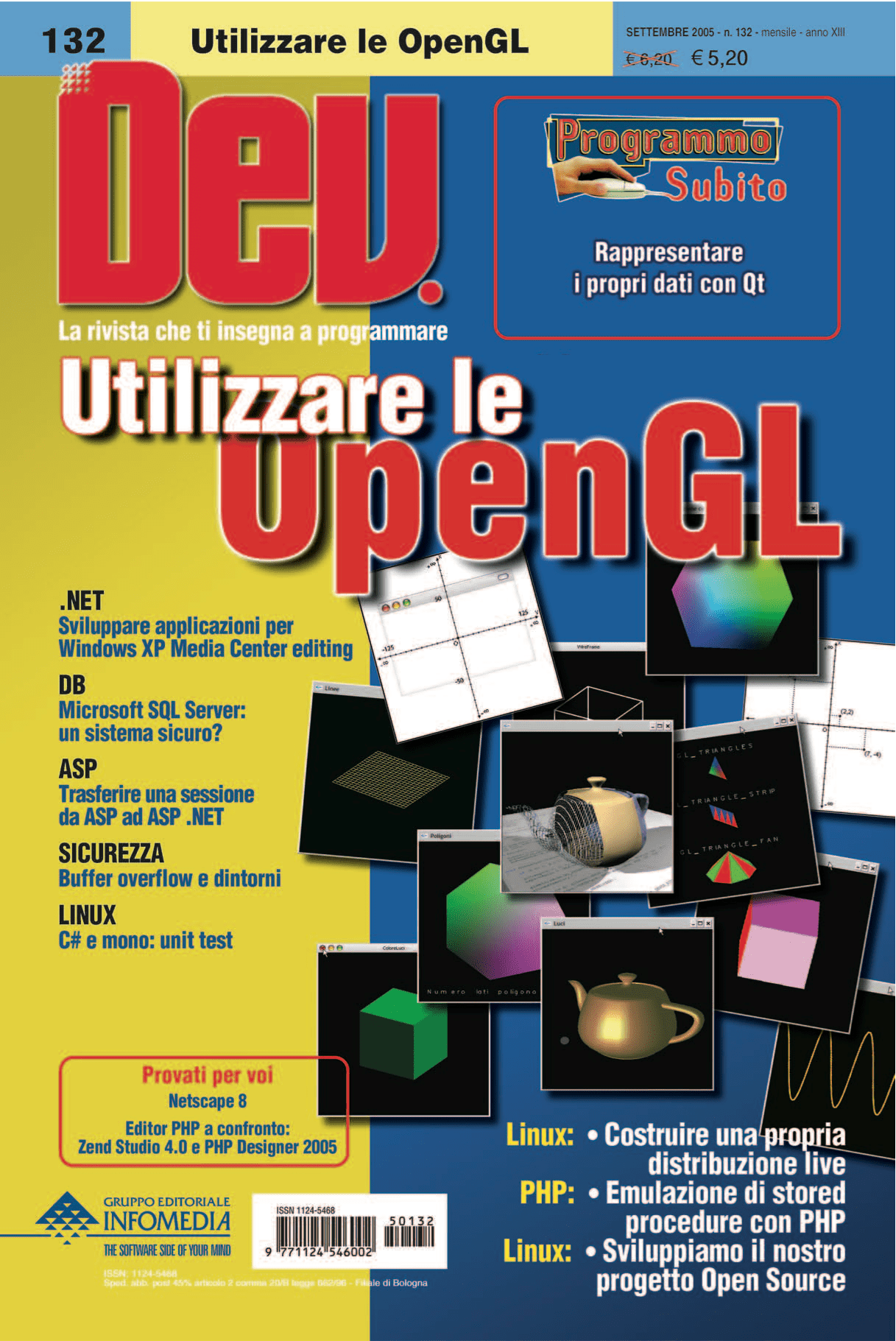We are finally ready to start our adventure into the world of 3D graphics. We will begin by the use of the most simple cases up to the geometric transformation of objects.

## Introduction

Now that we have a code template to use for quickly writing new programs, we can delve into the world of 3D and get to know the basic functionality of OpenGL. I highly recommend to you to study and compile the source code supplied with the articles and also make every change you have in mind to get more complex results.

## Working with primitives

The scenes, but in particular the objects that move inside them, are constituted by a composition of two-dimensional elementary shapes called primitives. We can compare with the bricks which, properly combined and aggregated together, give rise to all the more complex constructions imaginable.

Take the cube as a sample object. It consists of six faces, each of which may be drawn by a quadrilateral-type primitive or, dividing it into two equal parts, by two triangles.

OpenGL can work with several primitive shapes, from simple points to polygons. In total there are 10 different types, summarized below

 GL_POINTS Simple dots GL_LINES Lines GL_LINE_STRIP Sequence of lines GL_LINE_LOOP Closed sequence of lines GL_TRIANGLES Triangles GL_TRIANGLE_STRIP Sequence of triangles GL_TRIANGLE_FAN Fan sequence of triangles GL_QUADS Quadrilaterals GL_QUAD_STRIP Sequence of quadrilaterals GL_POLYGON Polygons

But which method is adopted to draw these figures in space? The “lowest common denominator” for all the primitives is the vertex, that stands for a defined point in space represented by its coordinates x, y, z. The more used OpenGL function to specify a vertex is

``glVertex3f(GLfloat x, GLfloat y, GLfloat z)``

Consequently, a primitive is nothing more than an interpretation of a list of vertices. Now that we know how to indicate the coordinates of points in space, we are able to take into consideration each of the types of primitives.

Keep in mind that the coordinates system of the window is different from that used by OpenGL, so the respective axes of y are reversed. For this reason while in the first case the positive values ​​are downwards oriented, in the second they start from the bottom and should go upwards.

## Points

Let’s take a look to the following code

`````` glBegin(GL_POINTS);
glVertex3f(0.0f, 0.0f, 0.0f);
glEnd();``````

We observe that the function calls that describe the vertices must be enclosed between the pair glBegin()/glEnd(), so setting the appropriate parameters OpenGL will interpret them by drawing the desired figure. In this case we had to draw GL_POINTS simple points.

You might be tempted to think that if you have to draw more than one figure is needed a glBegin/glEnd pair for each. This would only produce significant delays during the execution of the program. OpenGL, in fact, because of the parameter passed to glBegin can realize how many vertices are needed to describe the figure. In fact, writing

``````glBegin(GL_POINTS);
glVertex3f(0.0f, 0.0f, 0.0f);
glVertex3f(10.0f, 0.0f, 0.0f);
glVertex3f(5.0f, 10.0f, 0.0f);
glEnd();``````

the three dots will be rendered properly, but not a triangle. In Figure 1, is shown the output produced by the sample program Punti.exe.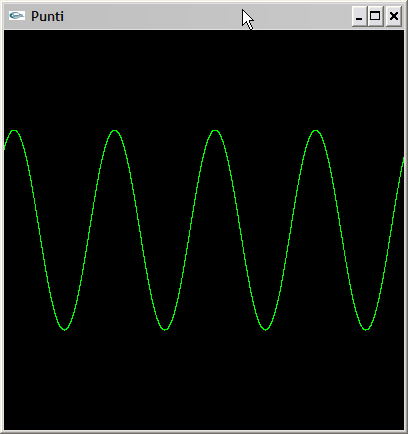Figure 1 – Image taken from Punti.exe program that shows how to create two-dimensional graphs by the primitive GL_POINTS.

## Lines

The next level after learning the use of individual points is to understand how to display lines: simple specifying two vertices one for the start position and another one for the end. Observe the following code

`````` glBegin(GL_LINES);
glVertex3f(-10.0f, 0.0f, 0.0f);
glVertex3f(10.0f, 0.0f, 0.0f);
glEnd();``````

which draws a horizontal line. If there is an incorrect number of vertices, for example an odd number, the surplus will simply be ignored.

Often when drawing figures with neighboring lines we could face a long sequence of vertices where many of them are duplicates. To draw a square with this system, for example, we would be forced to proceed in this way

`````` glBegin(GL_LINES);
glVertex3f(-10.0f, 0.0f, 0.0f);  // Side 1 point 1
glVertex3f(10.0f, 0.0f, 0.0f);   // Side 1 point 2
glVertex3f(10.0f, 0.0f, 0.0f);   // Side 2 point 1
glVertex3f(10.0f, 10.0f, 0.0f);  // Side 2, item 2
glVertex3f(10.0f, 10.0f, 0.0f);  // Side 3 point 1
glVertex3f(-10.0f, 10.0f, 0.0f); // Side 2 point 3
glVertex3f(-10.0f, 10.0f, 0.0f); // Side 1 point 4
glVertex3f(-10.0f, 0.0f, 0.0f);  // Side 4 point 2
glEnd();``````

Notice that we indicated 8 vertices, twice as necessary. Using the parameter of GL_LINE_STRIP with glBegin(), is no longer necessary to specify the vertices in common between two different lines. We will only provide a list of vertices and OpenGL will connect them drawing a classical broken line. Observe how in the following code all other vertices are concatenated together

``````glBegin(GL_LINE_STRIP);
glVertex3f(-10.0f, 0.0f, 0.0f);  // Side 1 point 1
glVertex3f(10.0f, 0.0f, 0.0f);   // Side 1 point 2 - point 1 Side 2
glVertex3f(10.0f, 10.0f, 0.0f);  // Side 2 point 2 - point 3 Side 1
glVertex3f(-10.0f, 10.0f, 0.0f); // Side 3 point 2 - point 4 Side 1
glVertex3f(-10.0f, 0.0f, 0.0f);  // Side 4 point 2
glEnd();``````

Now it took only 5 vertices. A great saving of time and data to be transferred from computer main memory to the accelerator board. But we can do better: have you noticed that the first and last vertices are identical? GL_LINE_LOOP, in fact, behaves similarly to the previous one apart from the fact that a line is drawn between the last and the first vertex specified. In terms of code you have

``````glBegin(GL_LINE_LOOP);
glVertex3f(-10.0f, 0.0f, 0.0f);  // Side 1 point 1
glVertex3f(10.0f, 0.0f, 0.0f);   // Side 1 point 2 - point 1 Side 2
glVertex3f(10.0f, 10.0f, 0.0f);  // Side 2 point 2 - point 3 Side 1
glVertex3f(-10.0f, 10.0f, 0.0f); // Side 3 point 2 - Side 4 point
glEnd();``````

An example of use of the lines capabilities, produced by the program Linee.exe, is visible in Figure 2.

## Triangles

With the lines you can draw any kind of 3D object. It is immediate to observe that the figures are created with this technique are not solid, but have an appearance that is normally called ‘wire-frame’. For this reason we will mainly use polygons, or closed figures, because they can be filled with a selected color.

The first polygon that we consider is the triangle which, consisting of only three sides, is the most simple shape possible. Also mind that OpenGL is particularly optimized to work with the triangles therefore this choice is the most usually adopted for solids construction.

The parameter GL_TRIANGLES draws, of course, a triangle connecting three vertices together as in

``````glBegin(GL_TRIANGLES);
glVertex3f(-10.0f, 0.0f, 0.0f);
glVertex3f(0.0f, 10.0f, 0.0f);
glVertex3f(10.0f, 0.0f, 0.0f);
glEnd();``````

Working in a three-dimensional space we must pay particular attention to the fact that every polygon can be seen from different angles. For this reason we can say that it has, in reality, two faces: the front face and the rear one. But how to discern between the two? The method adopted by OpenGL is to distinguish the order in which you specify the polygon’s vertices. Thus, the difference arises from the order of readed vertices and can occur that they are listed clockwise – Clockwise winding – or counterclockwise – Counterclockwise winding.

By default OpenGL considers the vertices oriented in an counterclockwise direction as the front face of the polygon. Through the function below is possible to specify the backface for the vertices indicate before

``glFrontFace(GL_CW)``

The importance of being able to make this distinction will be clear later in this article when we will discuss about removing the hidden faces. In the box previously showed we can see that there are other parameters that glBegin() accepts to draw triangles: GL_TRIANGLE_STRIP and GL_TRIANGLE_FAN.

With the first of the two can generate chains of adjacent triangles which save time and amount of data transferred, in the same way that works for the lines. The mechanism of construction of the chain consists in considering the last two vertices specified as the first two of the next triangle. In this way the next vertex will be taken as the final one of the triangle to be drawn. Look at an example

``````glBegin(GL_TRIANGLE_STRIP);
glVertex3f(-10.0f, 0.0f, 0.0f); // ​​Triangle 1 vertex 1
glVertex3f(0.0f, 10.0f, 0.0f);  // ​​Triangle 1 vertex 2 - Vertex 1 Triangle 2
glVertex3f(10.0f, 0.0f, 0.0f);  // ​​Triangle 1 vertex 3 - Triangle 2 vertex 2
glVertex3f(30.0f, 10.0f, 0.0f); // ​​Triangle 2 vertex
glEnd();``````

The GL_TRIANGLE_FAN parameter produces a group of triangles connected to a central point. In this case the first vertex specified will be considered as the central. Every other vertex is connected to the previous to form the new triangle, as in the following

``````glBegin(GL_TRIANGLE_FAN);
glVertex3f(-10.0f, 0.0f, 0.0f); // Central vertex
glVertex3f(0.0f, 10.0f, 0.0f);  // ​​Triangle 1 vertex 2
glVertex3f(10.0f, 0.0f, 0.0f);  // ​​Triangle 1 vertex 3 - Triangle 2 vertex 2
glVertex3f(30.0f, 10.0f, 0.0f); // ​​Triangle 2 vertex 3 - Triangle 3 vertex 2
...
glEnd ();``````

The example program Triangoli.exe whose output is presented in Figure 3 shows various examples of triangles.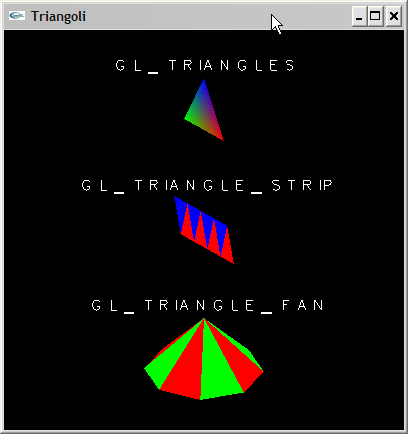Figure 3 – In this picture we see an example about each of the three primitive types GL_TRIANGLEXXX

By now we should have understood the functioning of the mechanism that regulates the creation of primitives. We can therefore afford to spend a few words about the quadrilaterals which are simply a list of four vertices, known as GL_QUADS by OpenGL.

The only precaution to follow is that these vertices must lie on the same plane. As for the triangles is possible to create chains of quadrilaterals through GL_QUAD_STRIP, where each next of them is indicated by the last two vertices of the quadrilateral previous joined to two other new vertices. We clarify everything with the usual example, this time building a cube

``````glBegin(GL_QUADS);
// Upper side
glVertex3f(-10.0f, 10.0f, 10.0f);
glVertex3f(-10.0f, 10.0f,-10.0f);
glVertex3f(10.0f, 10.0f,-10.0f);
glVertex3f(10.0f, 10.0f, 10.0f);

// Lower side
glVertex3f(-10.0f,-10.0f, 10.0f);
glVertex3f(-10.0f,-10.0f,-10.0f);
glVertex3f(10.0f,-10.0f,-10.0f);
glVertex3f(10.0f,-10.0f, 10.0f);
glEnd();

// Lateral faces
glVertex3f(-10.0f,-10.0f, 10.0f); // Quad 1 - 1 Vertex
glVertex3f(-10.0f, 10.0f, 10.0f); // Quad 1 - 2 Vertex
glVertex3f(10.0f,-10.0f, 10.0f);  // Quad 1 - 3 Vertex
glVertex3f(10.0f, 10.0f, 10.0f);  // Quad 1 - 4 Vertex
glVertex3f(10.0f,-10.0f,-10.0f);  // Quad 2 - 3 Vertex
glVertex3f(10.0f, 10.0f,-10.0f);  // Quad 2 - 4 Vertex

glVertex3f(-10.0f,-10.0f,-10.0f); // Quad 3 - Vertex 3
glVertex3f(-10.0f, 10.0f,-10.0f); // Quad 3 - 4 Vertex

glVertex3f(-10.0f,-10.0f, 10.0f); // Quad 4 - Vertex 3
glVertex3f(-10.0f, 10.0f, 10.0f); // Quad 4 - 4 Vertex
glEnd();``````

In Figure 4 there is the output of the program Quadrilateri.exe.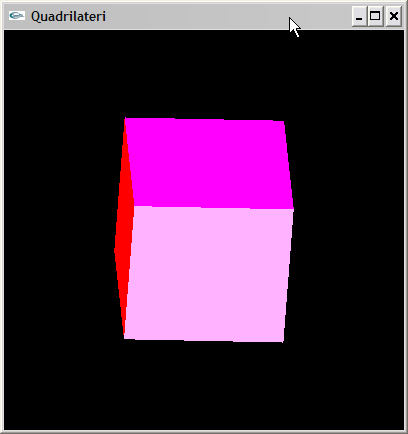Figure 4 – Here we have built a cube using quadrilaterals. In particular we use the primitive GL_QUADS for the upper and lower faces, while GL_QUAD_STRIP for the lateral.

## Polygons

The final primitive known from OpenGL is GL_POLYGON which is a figure composed by any number of vertices. The restrictions, or rules, of polygon construction are two. The first wants, as for the quadrilaterals, that all the vertices of the polygon must lie on the same plane while the second consists in the fact that a polygon must necessarily be convex, ie should not result in no case that drawing line any that intersects the polygon, in more than two points.

The program Poligoni.exe produces the output shown in Figure 5.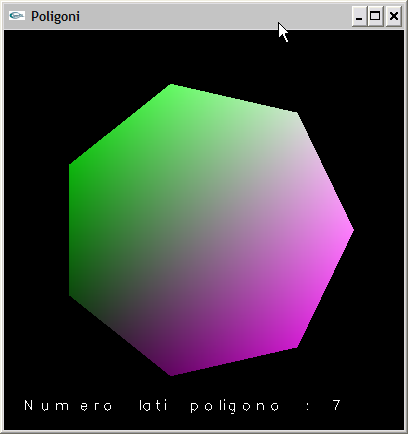Figure 5 – In this picture we see a simple example of primitive GL_POLYGON

## Back faces culling

As already said, when displaying a polygon in space it happens that OpenGL should draw both faces, the front and rear. In the last example presented we have built a solid, ie, an object with three dimensions, as a composition of two-dimensional primitives. Because each polygon has a face, that being “inside” the cube, does not appear in any case, so by setting this flag

``glEnable(GL_CULL_FACE)``

and launching the function

`` glCullFace (GL_BACK)``

we obtain a considerable saving of time asking OpenGL to not draw at all the back faces of the polygons.The glCullFace() takes as parameters the values GL_BACK, GL_FRONT GL_FRONT_AND_BACK – although I think the latter is useful in very few cases only. At any time, even within glBegin/glEnd calls, we can change the settings or disable this feature using

``glDisable(GL_CULL_FACE)``

Look at the example FacceNascoste.exe to clarify further doubts.

## Coloring

We have already encountered the function glColor that allows you to set the current drawing color. Through this you can create beautiful effects using a very interesting feature of OpenGL that is called Shading.

For each vertex of a primitive, in fact, we can set its own color. The figure will be drawn and filled with a gradient of colors interpolated between the color of a vertex to others specified. Figure 6 shows a cube formed by quadrilaterals stained with this technique.

By default, the color interpolation is enabled, but you can change it at any time with

``glShadeModel(GL_FLAT)``

In this way while drawing the polygon, will be taken into account only the last occurrence of the function glColor. With the call

``glShadeModel(GL_SMOOTH)``

everything returns to normality.

## Depth test

Look at Figure 7, can you notice something strange? The green plane on which should be placed the red cube appears in front of it making the image a little unreal. This happens because the code firstly asked to draw the cube and only after the plan. OpenGL draws the figures one after the other by overwriting the image below.Figure 7 – The picture shows a situation where it is not used the depth control for objects

The control of depth makes it possible to eliminate this problem and is able to establish the effective depth of an object and its actual location among the others, within the scene. To activate the depth test are needed the following steps. First we must ask GLUT to reserve memory for the required buffer, so we add the flag GLUT_DEPTH to the initialization function of the display as in

``glutInitDisplayMode(GLUT_DOUBLE | GLUT_RGBA | GLUT_DEPTH)``

then we must make sure to clear the buffer used for depth values before we start drawing one frame. For this purpose we make use of the call to glutClear() already present in the function RenderScene(), which will have to write in this form

``glutClear(GL_COLOR_BUFFER_BIT | GL_DEPTH_BUFFER_BIT)``

Finally we must enable the depth test. It can be done wherever necessary, but for our purposes is just fine in SetupRC() function where we put the line

``glEnable(GL_DEPTH_TEST)``

## Transformations

When we think about 3D graphic we should know that it is composed of three-dimensional objects, but all we see on screen is nothing more than a two dimensional image. There is called projection, in fact, a complex process that transforms the 3D objects data into 2D image representation.

There are also other types of transformations that produce different effects, you can: rotate, move or resize objects. Each type of geometric transformation is applied to the scene at different times since the vertices are specified until they appear on the screen.

In the first phase is applied a transformation to the ‘view’ – Viewing Transformations – used to determine the point of view of the scene. The OpenGL command to set this point is

``````gluLookAt (GLdouble eyex, eyey GLdouble, GLdouble eyez,
GLdouble centerx, GLdouble centery, GLdouble centerz, GLdouble upx, GLdouble upy, GLdouble upz);``````

The parameters of this function are intuitive, in fact, eye indicate the point where the observer is located, the center coordinates represent the point which is opposite to the observer, while up represents a vector pointing upwards, from the perspective of the observer. Anyone with some perplexity regarding the last parameter can think that is not sufficient to establish the position of an observer and the direction where him/her is watching. It seems also necessary ìto know the inclination of his head.

Without this information, in fact, we have no way of knowing whether it is watching, maybe, with your head upside down or 45 degrees tilted. The reason for which it is applied before the other lies in the fact that the displacement of the observation point determines a change in the working coordinate system. Any other change must take account of modifications made by this.

For example, we see an object centered at coordinates (0,0,0) from a distance, then we write

`````` gluLookAt (0.0f, 50.0f, 50.0f,     // ​​Position of the observation point
0.0f, 0.0f, 0.0f,       // ​​point to which "we turn our
// glance"
0.0f, 1.0f, 0.0f),      // ​​vector pointing upwards,
// perpendicularly to the plane``````

The example PuntoOsservazione.exe shows how to use this transformation to walk around the scene looking at the center of it.

The second type of transformations are the those that are applied to models – Modeling Transformations – mainly used to move objects in space, resize or rotate around the axis. Starting from the translations the function is

``glTranslatef(GLfloat x, GLfloat y, GLfloat z)``

that generates the movement of the object by the specified entity, for each axis. We then, for rotations can use

``glRotatef(GLfloat angle, GLfloat x, GLfloat y, GLfloat z)``

which rotates the object by an angle equal expressed in degrees. To indicate around which given axis must be rotated an object we must pass the 1.0f value to the parameters x, y or z. In the simplest case a rotation takes place around a single axis, but it is possible to realize rotations involving all axes. In the exampleglRotatef (GLfloat angle, GLfloat x, GLfloat y, GLfloat z)an object would be rotated around to the Z axis

``glRotatef(45, 0.0f, 0.0f, 1.0f)``

The scaling works by increasing or decreasing the size of the objects expanding the vertices, along the three axes. Each parameter of the function

``glScalef(GLfloat x, GLfloat y, GLfloat z)``

represents the multiplication factor for the object size. A value of 1.0f leaves unaltered the size along the axis, a larger value causes an increase, while a value tending to zero determines the shrinkage. Writing

``glScalef(1.0f, 4.0f, 1.0f)``

any object would be stretched vertically, but would keep intact the other dimensions.

We should pay particular attention to the fact that the described transformations are not applied to the single object, but to the entire reference system, so any other further operation will be affected by the new settings.

Changing order of two transformations, for example a translation and a rotation, produces different effects as is done in this case

`````` glTranslatef (10.0f, 0.0f, 0.0f);
glRotatef (45, 0.0f, 0.0f, 1.0f);
...
drawCube();``````

and

`````` glRotatef (45, 0.0f, 0.0f, 1.0f);
glTranslatef (10.0f, 0.0f, 0.0f);
...
drawCube();``````

In the first case we have moved the cube of 10 units on the X axis with a subsequent rotation of 45 degrees on the Z axis. In the second the opposite has occurred, ie, was first carried out a rotation of 45 degrees along the Z axis and then a translational motion along the X axis. Can you notice the difference?

Let’s move on to the next type of transformations: the projection transformations. This type of transformation is used to define which parts are visible to the viewer of 3D space and specify how the 3D scene is projected on the screen. There are two types of projection

• The Orthogonal projection, which does not discern any difference on the objects distance. They, in fact, will have the same size if they are near to the observation point or not. We also said that this type of projection is mostly used in CAD applications, or in video games, to create menus or 2D images.
• The Perspective projection which produces images that mirror the optical phenomenon where identical objects distant from each other seems to have different sizes or where parallel lines seem to converge away from the observer.

The perspective projection is activated with the function

``gluPerspective (GLdouble fovy, GLdouble aspect, GLdouble zNear, GLdouble zFar)``

We give a brief explanation of the parameters. The first and most cryptic is ‘fovy’ the angle of the visual field in the vertical direction, ‘aspect’ is the usual aspect ratio ie the ratio between width and height of the window. Finally zNear and zFar are respectively the visible boundary closer to the viewer and the farther away.

The last type of transformation is what happens to the viewport when the final image is mapped physically on the window. However, the user does not need to deal with this aspect.

## Conclusions

After reading this article you will become familiar with the OpenGL drawing functions. You can now create your own fully functional program that displays various types of solid objects and not.

In the next article I will conclude this process by studying ways to create more complex scenes and use advanced features such as texture mapping, lighting effects and more.

## Bibliography

1. Richard S.Wright jr. and Benjamin Lipchak, “OpenGL SuperBible – Third Edition”, SAMS, 2005, ISBN 0-672-32601-9